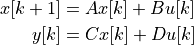# control.matlab.markov¶

control.matlab.markov(Y, U, m=None, transpose=False)[source]

Calculate the first m Markov parameters [D CB CAB …] from input U, output Y.

This function computes the Markov parameters for a discrete time systemgiven data for u and y. The algorithm assumes that that C A^k B = 0 for k > m-2 (see ). Note that the problem is ill-posed if the length of the input data is less than the desired number of Markov parameters (a warning message is generated in this case).

Parameters
• Y (array_like) – Output data. If the array is 1D, the system is assumed to be single input. If the array is 2D and transpose=False, the columns of Y are taken as time points, otherwise the rows of Y are taken as time points.

• U (array_like) – Input data, arranged in the same way as Y.

• m (int, optional) – Number of Markov parameters to output. Defaults to len(U).

• transpose (bool, optional) – Assume that input data is transposed relative to the standard Time series data. Default value is False.

Returns

H – First m Markov parameters, [D CB CAB …]

Return type

ndarray

References

1

J.-N. Juang, M. Phan, L. G. Horta, and R. W. Longman, Identification of observer/Kalman filter Markov parameters - Theory and experiments. Journal of Guidance Control and Dynamics, 16(2), 320-329, 2012. http://doi.org/10.2514/3.21006

Notes

Currently only works for SISO systems.

This function does not currently comply with the Python Control Library Time series data for representation of time series data. Use transpose=False to make use of the standard convention (this will be updated in a future release).

Examples

```>>> T = np.linspace(0, 10, 100)
>>> U = np.ones((1, 100))
>>> T, Y = ct.forced_response(ct.tf(, [1, 0.5], True), T, U)
>>> H = ct.markov(Y, U, 3, transpose=False)
```